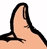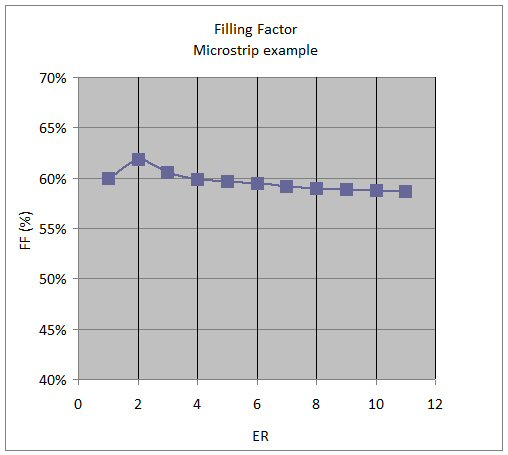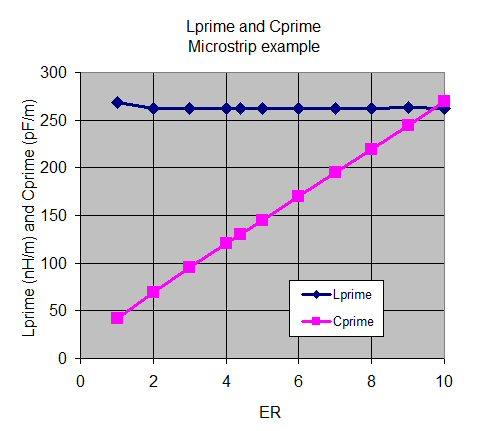# Filling Factor

New for August 2010! Filling factor is what connects Keffective to ER. Filling factor is a measure of the percentage of the electric fields in a transmission line that cut through the substrate.

In non-TEM transmission lines such as CPW and microstrip, the electric fields cut through two (or sometimes more) dielectric materials. For now, we will only consider the case of two materials, and one of them is air (ER=1).

If FF is the percentage of fields in the material, then 1-FF is the percentage of fields in air.

Keff=ER*FF+1x(1-FF)

Solving for FF,

FF=(Keff-1)/(ER-1)

In most calculations, engineers are only interested in Z0 and Keff. But they miss what is really going on by ignoring FF. Let's start by stating a Microwaves101 Rule of Thumb, and then back it up by way of example:

### Microstrip Filling Factor Rule of ThumbFor a given microstrip or stripline geometry, the filling factor is very nearly a constant versus the value of the dielectric constant of the substrate. The inductance per length does not change versus the dielectric constant of the substrate, only the capacitance/length does.

### Microstrip filling factor example

Let's look at an example geometry. Suppose you were evaluating an unknown board material, with transmission line of the following microstrip geometry:

H=2.7 mils

W=5.0 mils

T=2 mils

Let's look at the filling factor, for any dielectric constant ER from 1.01 to 10 (we can't use ER=1, because it results in divide-by-zero situation!)

We used ADS to calculate the Keff and Z0 for all of these dielectrics, and came up with the following table:

ER Keff Z0 FF
1.01 1.006 79.9 0.600
2 1.619 61.4 0.619
3 2.212 52.5 0.606
4 2.796 46.7 0.599
5 3.377 42.5 0.594
6 3.958 39.3 0.592
7 4.539 36.7 0.590
8 5.120 34.6 0.589
9 5.702 33.8 0.588
10 6.285 31.2 0.587

A note about calculating Keff... you could use the Microwaves101 microstrip calculator, and come up with values of filling factor. But we'll let you in on a secret... our microstrip calculator is not as accurate as ADS, because we used simplified equations that don't take into account thickness of the metal strip. In the case of this geometry, the error is large enough so that we decided to go with the better calculation, indeed, the Microwaves101 Keff value is almost 10% too high. In the future, we'll try to post a calculator with the full microstrip solution, but for now, let's just say beware of free calculators on the web!

As you can see from the table, FF pretty much remains constant with regard to ER. Let's look at a plot, a picture is worth a thousand words:Now let's calculate the inductance and capacitance per length (L' and C'), and plot them.Guess what? Inductance/length doesn't change much, when you radically change the dielectric ER in a non-TEM transmission line. The capacitance/length changes linearly, as you'd expect. This is a direct result of FF remaining constant versus ER!

###CPW Filling Factor Rule of Thumb

For ideal coplanar waveguide (with very thick substrate and no ground plane on the back side, thin, perfect conductors), filling factor is 50%. Therefore the Keff is equal to:

Keff=(ER+1)/2

This is the average value of air (ER=1) and the substrate.

Author : Unknown Editor